#### Finding quaternion parameters from a rotation matrix

Recall from Section 3.2.3 that given a rotation matrix (3.43), the yaw, pitch, and roll parameters could be directly determined using the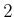function. It turns out that the quaternion representation can also be determined directly from the matrix. This is the inverse of the function in (4.20).4.9

For a given rotation matrix (3.43), the quaternion parameters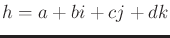can be computed as follows . The first component is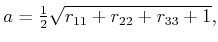(4.24)

and if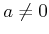, then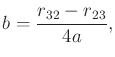(4.25)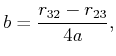(4.26)

and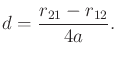(4.27)

If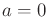, then the previously mentioned equator problem occurs. In this case,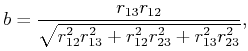(4.28)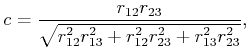(4.29)

and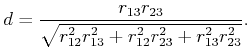(4.30)

This method fails if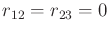or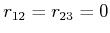or. These correspond precisely to the cases in which the rotation matrix is a yaw, (3.39), pitch, (3.40), or roll, (3.41), which can be detected in advance.

Steven M LaValle 2020-08-14